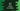# 3 different Java program to find the volume of a cylinder## Java program to find the volume of a cylinder:

In this post, we will learn how to find the volume of a cylinder in Java. We will learn different ways to do the calculation. We need the height and radius of a cylinder to calculate its volume. The program will take these values as inputs from the user, calculate the volume of that cylinder and print the value.

### How to find the volume of a cylinder:

To find the volume of a cylinder, we need its height and radius. Once we get these values, we can find the volume of that cylinder with the below formula:

``π * r * r * h``

Where π is the mathematical constant PI, r is the radius of the cylinder and h is the height of the cylinder.

The program will take the radius and height of the cylinder as inputs from the user and calculate the volume of the cylinder with the above formula.

### Method 1: Basic program to find the volume of a cylinder:

Let’s take a look at the below program:

``````class Main {

public static void main(String[] args) {
double radius = 40, height = 28;
double PI = 3.1415926;

System.out.println("Volume of the cylinder is: " + volume);
}
}``````

Here, the value of the radius, height, **and PI are predefined. All of these are predefined values and the volume is calculated by using the above formula.

It will print the below output:

``Volume of the cylinder is: 140743.34848000002``

### Method 2: Example to find the volume of a cylinder by taking the values as inputs from the user:

The above program uses predefined values. We can also take these values as inputs from the user. The value of PI is already defined in the Math class. We can use it instead of creating another double value. Let’s write down the program:

``````import java.util.Scanner;

class Main {

public static void main(String[] args) {
Scanner sc = new Scanner(System.in);
double PI = Math.PI;

System.out.println("Enter the radius of the cylinder: ");

System.out.println("Enter the height of the cylinder: ");
height = sc.nextDouble();

System.out.println("Volume of the cylinder is: " + volume);
}
}``````

In the above program, we are reading the radius and height values as inputs from the user. A scanner object is used to read the user-input values. The PI value is used from Math.PI. The volume is calculated as per the above formula.

If you run this program, it will print the output as below:

``````Enter the radius of the cylinder:
30
Enter the height of the cylinder:
40
Volume of the cylinder is: 113097.33552923254``````

### Method 3: Example to find the volume of a cylinder by using a separate method:

We can use a separate method to calculate the volume of the cylinder. The advantage of using a separate method is that we can call this method from different classes. It will take the radius and height as parameters and return the volume of the cylinder.

Below is the program:

``````import java.util.Scanner;

import static java.lang.Math.PI;

class Main {
public static double getCylinderVolume(double radius, double height) {
}

public static void main(String[] args) {
Scanner sc = new Scanner(System.in);

System.out.println("Enter the radius of the cylinder: ");

System.out.println("Enter the height of the cylinder: ");
height = sc.nextDouble();

System.out.println("Volume of the cylinder is: " + volume);
}
}``````

Here,

• getCylinderVolume method is used to get the volume of the cylinder. It takes the radius and height as the parameters and returns the volume. The returned value is stored in the variable volume. The last line is printing the volume value.

It will give similar results:

``````Enter the radius of the cylinder:
30
Enter the height of the cylinder:
40
Volume of the cylinder is: 113097.33552923254``````